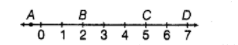# Which letter best represents the location ofQuestion:

Which letter best represents the location of $\sqrt{25}$ on a number line?(a) $A$

(b) $B$

(c) $C$

(d) $D$

Solution:

(c) We have, $\sqrt{25}=5$

Therefore, 5 at letter $C$ represents the best location of $\sqrt{25}$ on number line.